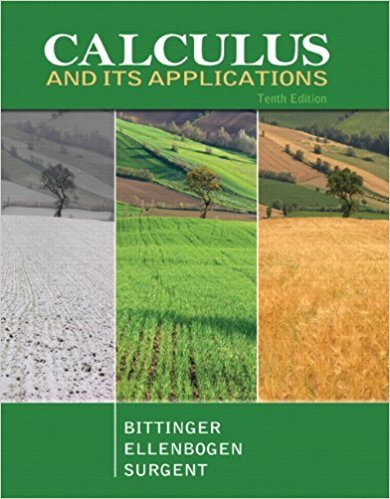×
×

# Solutions for Chapter 4.4: Properties of Definite Integrals## Full solutions for Calculus and Its Applications | 10th Edition

ISBN: 9780321694331Solutions for Chapter 4.4: Properties of Definite Integrals

Solutions for Chapter 4.4
4 5 0 294 Reviews
26
2
##### ISBN: 9780321694331

Chapter 4.4: Properties of Definite Integrals includes 76 full step-by-step solutions. This textbook survival guide was created for the textbook: Calculus and Its Applications, edition: 10. Since 76 problems in chapter 4.4: Properties of Definite Integrals have been answered, more than 26710 students have viewed full step-by-step solutions from this chapter. Calculus and Its Applications was written by and is associated to the ISBN: 9780321694331. This expansive textbook survival guide covers the following chapters and their solutions.

Key Calculus Terms and definitions covered in this textbook
• Arctangent function

See Inverse tangent function.

• Cone

See Right circular cone.

• Equilibrium point

A point where the supply curve and demand curve intersect. The corresponding price is the equilibrium price.

• Infinite discontinuity at x = a

limx:a + x a ƒ(x) = q6 or limx:a - ƒ(x) = q.

• Jump discontinuity at x a

limx:a - ƒ1x2 and limx:a + ƒ1x2 exist but are not equal

• Line of travel

The path along which an object travels

• Median (of a data set)

The middle number (or the mean of the two middle numbers) if the data are listed in order.

• Normal curve

The graph of ƒ(x) = e-x2/2

• Numerical model

A model determined by analyzing numbers or data in order to gain insight into a phenomenon, p. 64.

• Ordered pair

A pair of real numbers (x, y), p. 12.

• Parallelogram representation of vector addition

Geometric representation of vector addition using the parallelogram determined by the position vectors.

• Parametric equations

Equations of the form x = ƒ(t) and y = g(t) for all t in an interval I. The variable t is the parameter and I is the parameter interval.

• Quartic function

A degree 4 polynomial function.

• Richter scale

A logarithmic scale used in measuring the intensity of an earthquake.

• Right angle

A 90° angle.

• Secant line of ƒ

A line joining two points of the graph of ƒ.

• Term of a polynomial (function)

An expression of the form anxn in a polynomial (function).

• Vertex of an angle

See Angle.

• Xscl

The scale of the tick marks on the x-axis in a viewing window.

• Zero factor property

If ab = 0 , then either a = 0 or b = 0.

×